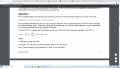# Sine wave look up table calculations does not match online generator

#### zazas321

Joined Nov 29, 2015
936
Hey. I am trying to learn DDS and how to create a varying frequency sine wave using DAC.I am using formula above to calculate sample values for my sine wave. In my case, I am using 1024 points and full scale is 4095.
When n=1, the calculated value is 25,126
when n=2, the calculated value is 50.25 (Assume zero value is 0)

I am checking my calculations with the sine look up table generator:And I can see that the value n is 2048 which is half of the maximum value 4095,
n is 2060 which is 12 higher then 2048, however, in my case I have calculated it to be 25,126. Any ideas why would that be?

Last edited:

#### zazas321

Joined Nov 29, 2015
936
It appears that the calculations I get are skipping a sample everytime I increment

#### kubeek

Joined Sep 20, 2005
5,791
What is your full scale voltage in your calculation? Should be 2047.

#### zazas321

Joined Nov 29, 2015
936
Why is it 2047? I am using 12 bit DAC which means that the full scale is (2^12 -1) = 4095 .
Is that not right?

#### kubeek

Joined Sep 20, 2005
5,791
That is not right. That is full scale of the ADC, but you want the center of the sine wave at 2048, so you have only 4095-2048=2047 points for it to go positive, and another 2048 points that it can go negative. So you need to multiply the sine by 2047 or less in order for the signal to not get limited.